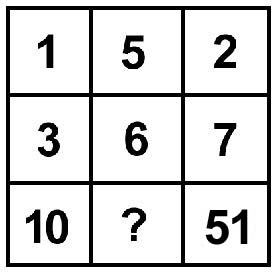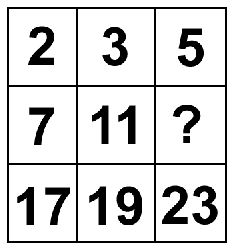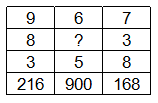0
2225

# RRB JE Number Series Questions PDF

Download RRB JE Number Series Questions and Answers PDF. Top 30 RRB JE Number Series questions based on asked questions in previous exam papers very important for the Railway JE exam.Question 1: Find the number which should replace ‘?’.
2, 3, 7, 25, ?

a) 121

b) 110

c) 100

d) 108

Question 2: Find the number which will replace ‘?’.
4, 18, 48, 100, ?

a) 200

b) 136

c) 152

d) 180

Question 3: Which of the following numbers replaces the question mark in the given sequence?
2, 4, 32, 576, ?

a) 1236
b) 1048
c) 894
d) None of theseQuestion 4: A series is given with one missing term. Choose the correct alternative from the given optons that will complete the series.
18, 26, 38, 62, ? , 102

a) 82

b) 74

c) 78

d) 68

Question 5: Identify the term which will come next in the following number series.
11, 15, 51, 87, 312, ?

a) 447

b) 348

c) 512

d) 391

Question 6: Find the next number in the series.
243, 486, 162, 324, 108, 216, ?

a) 432

b) 81

c) 72

d) 512

Question 7: A series is given with one missing term. Choose the correct alternative from the given optons that will complete the series.
1937, 2256, 2577, 2900, 3225, ?

a) 3552

b) 3486

c) 3628

d) 3494

Question 8: What number comes in the place of the question mark?a) 21

b) 31

c) 20

d) 41

Question 9: In the figure given below, find the number that can come in the place of the question mark?a) 13

b) 14

c) 15

d) 16Question 10: Find the missing number ?
11,13,17,19,23,25,29,31………..

a) 35

b) 47

c) 53

d) 51

Question 11: From the alternatives, select the set which is most like the given set. Given set (23,29,31)-

a) (17,21,29)

b) (31,37,49)

c) (13,15,23)

d) (41,43,47)

Question 12: Find the next number in the series:
1024, 512, 256, 128, 64, _____

a) 32

b) 36

c) 42

d) 16

Question 13: Find the missing number in the series.
51, ?, 46, 42, 37, 31, 24

a) 50

b) 49

c) 48

d) 102

Question 14: If “CLASS” is coded as “BKZRR” then how will “RIGHT” be coded?

a) QHFGS

b) RGEFT

c) SFDGU

d) VEGHW

Question 15: Complete the following series: 10, 17, 25, 34, 44, ?

a) 54

b) 55

c) 64

d) 61

Question 16: Find the median of the following numbers
14, 23, 20, 12, 11, 15, 24, 17, 9, 21, 25

a) 15

b) 20

c) 17

d) 11Question 17: How many 4’sare there in the following number – series which are preceded by 5 but NOT followed by 7 ?
23423425473243192547547234529546

a) Three

b) Four

c) Five

d) None

Question 18: Consider the natural number 88935. Then the least natural number by which we can divide or multiply the number to make it a square number is

a) 3

b) 5

c) 15

d) None of these

Question 19: Which number comes next in the series
1, 8, 15, 22, 29, 36, ?

a) 39

b) 49

c) 43

d) 50

Question 20: Identify the wrong number in the following series- 842, 790, 739, 686, 634, 582

a) 842

b) 739

c) 686

d) 634

Instructions

In the following question, select the missing number from the given series.

Question 21: 9, 19, 37, 75, 149, ?

a) 297

b) 298

c) 300

d) 299Question 22: In the following question, select the missing number from the given series.
54, 51, 48, 45, 42, ?

a) 38

b) 36

c) 40

d) 39

Question 23: A series is given with one missing term. Choose the correct alternative from the given options that will complete the series.
15, 21, 24, 30, 33, ?

a) 39

b) 43

c) 37

d) 41

Question 24: A series is given with one missing term. Choose the correct alternative from the given options that will complete the series.
795, 626, 482, 361, ?, 180

a) 261

b) 304

c) 245

d) 273

Question 25: In the following question, select the missing number from the given series.

8, 16, 43, 107, 232, ?

a) 342

b) 448

c) 248

d) 528

Question 26: In the following question, select the missing number from the given series.
43, 58, 88, 133, ?, 268

a) 193

b) 203

c) 163

d) 218

Question 27: Insert the correct missing number from the choices given below

a) 36

b) 15

c) 51

d) 69

Question 28: Select the missing number from the given responses :a) 30

b) 55

c) 60

d) 70

Question 29: If 1 + 4=9, 2 + 8=18, 3+6 = 15 then 7 + 8 = ?

a) 41

b) 23

c) 30

d) 32

Question 30: 40% of 1620 + 30% of 960 = ? % of 5200

a) 12

b) 24

c) 16

d) 18The series follows the following pattern:
1! + 1 = 2
2! + 1 = 3
3! + 1 = 7
4! + 1 = 25
5! + 1 = 121

The series follows the following pattern – $n^3 – n^2$

$2^3 – 2^2 = 4$
$3^3 – 3^2 = 18$
$4^3 – 4^2 = 48$
$5^3 – 5^2 = 100$
$6^3 – 6^2 = 180$

4 = 2*1*2
32 = 4*2*4
576 = 32*3*6
Therefore, the next number in the sequence should be 576*4*8 = 18432.
Since there is no such option, the correct option to choose is D.

18 + 1*8 = 26
26 + 6*2 = 38
38 + 3*8 = 62
62 + 6*2 = 74
74 + 7*4 = 102
Hence, option B is the right answer.

The pattern which is being followed is that the square of the sum of the digits of the number is being added to the number to get the next number. For example, $11 + 2^2 = 15$
$15 + 6^2 = 51$
$51 + 6^2 = 87$
$87 + 15^2 = 312$
Hence, using the same logic the next term will be
$312 + (3+1+2)^2 = 348$
Hence, option B is the correct answer.

The series follows the following pattern:
243
243 * 2 = 486
486 /3 = 162
162 * 2 = 324
324/3 = 108
108 * 2 = 216
216 / 3 = 72

2256 – 1937 = 319
2577 – 2256 = 319 + 2
2900 – 2577 = 319 + 4
3225 – 2900 = 319 + 6
? = 3225 + 319 + 8 = 3552
Hence, option A is the right answer.

In the left column, 1 + $3^2$ = 10
In the right column, 2 + $7^2$ = 51
So, the middle column = 5 * $6^2$ = 41

The given list is the list of prime numbers from 2 to 23. So, 13 comes in the place of the question mark.

The difference between the numbers alternates between 2 and 4. For example, the first number is 2 less than the second number. And the second number is 4 less than the third and the third number is 2 less than the fourth and so on.

The numbers in the given set are prime numbers.Similarly, the numbers in the set of option D are also prime.

We see that the differences between consecutives terms is -512, -256, -128, -64. Hence, each term is half of the previous term.

Thus next term = 64/2 = 32.We see that the differences between consecutives terms is -4, -5, -6, -7. Thus, the differences are in arithmetic progression. Thus, the previous difference would have been -4+1=-3 and -3+1=-2. Thus, the term missing is 2 lesser than 51 and 3 greater than 46 i.e. 51-2=49.

In the coded language every letter is 1 back from the original word in alphabetical series. Hence, “Right” will be coded as “QHFGS”

10+7 = 17; 17+8 = 25 ; 25+9 = 34 ; 34+10 = 44 ; 44+11 = 55

To find the median, we have the arrange the numbers in the ascending order and then pick the number that’s at the centre of the list. So,

the list in arranging order is: 9, 11, 12, 14, 15, 17, 20, 21, 23, 24, 25. Since 17 is at the centre of the list, it is the median of the group.

There are 3 4s that are preceeded by 5s but are also followed by 7s. So, the answer is “none”.

88935=11$^{2}$×7$^{2}$×15

Thus, if we multiply or divide with 15 we get square numbers.

Each term is 7 greater than the previous term. Hence, the next number should be 36+7 = 43.

842 – 52 = 790
790 – 52 = 738
738 – 52 = 686
686 – 52 = 634
634 – 52 = 582
So, 739 is the wrong number in the series. It should be replaced with 738.

The pattern followed is :

9 $\times$ $2+1$ = 19

19 $\times$ $2-1$ = 37

37 $\times$ $2+1$ = 75

75 $\times$ $2-1$ = 149

149 $\times$ $2+1$ = 299

=> Ans – (D)

3 is subtracted from all the numbers.

54 – 3 = 51

51 – 3 = 48

48 – 3 = 45

45 – 3 = 42

42 – 3 = 39

=> Ans – (D)

15 + 1 + 5 = 21
21 + 2 + 1 = 24
24 + 2 + 4 = 30
30 + 3 + 0 = 33
33 + 3 + 3 = 39
Hence, option A is the right answer.

$795 – 13^2 = 626$
$626 – 12^2 = 482$
$482 – 11^2 = 361$
$361- 10^2 = 261$
$261 – 9^2 = 180$
Hence, option A is the right answer.

Cubes of consecutive integers are added.

8 $+(2)^3$ = 16

16 $+(3)^3$ = 43

43 $+(4)^3$ = 107

107 $+(5)^3$ = 232

232 $+(6)^3$ = 448

=> Ans – (B)

43 + 15 = 58

58 + 30 = 88

88 + 45 = 133

133 + 60 = 193

193 + 75 = 268

=> Ans – (A)

Value in row 1 $\times$ value in row 2 $\times$ Value in Row 3 = Value in Row 4

Let the unknown be $x$.
$6 \times x \times 5 = 900$
Hence $x$ = $\frac{900}{30}$
$x$ =$30$

Hence Option A is the correct answer

Here we are given :

1 + 4=9

2 + 8=18

3+6 = 15

So if the number is , x + y then it is equal to [(x+y) + y]

here we need to calculate value of 7 + 8 = [(7 + 8) + 8] = 23

40% of 1620 = $\frac{40 \times 1620}{100}$ = $648$
30% of 960 = $\frac{30 \times 960}{100}$ = $288$
Let the unknown percentage be $x$.
$936 = x \% of 5200$
$x = \frac{936 \times 100}{5200} = 18$